# Motion in One Dimension: JEE Main(Part- 2) - Physics, Solution by DC Pandey Notes | Study DC Pandey Solutions for JEE Physics - JEE

## JEE: Motion in One Dimension: JEE Main(Part- 2) - Physics, Solution by DC Pandey Notes | Study DC Pandey Solutions for JEE Physics - JEE

The document Motion in One Dimension: JEE Main(Part- 2) - Physics, Solution by DC Pandey Notes | Study DC Pandey Solutions for JEE Physics - JEE is a part of the JEE Course DC Pandey Solutions for JEE Physics.
All you need of JEE at this link: JEE

Non-uniform Acceleration

Ques 21: x-coordinate of a particle moving along this axis is: x = (2 + t2 + 2/3). Here, x is in metre and t in seconds. Find:
(a) position of particle from where it started its journey,
(b) initial velocity of particle and
(c) acceleration of particle at t = 2s.

Ans: (a) x = 2.0 m
(b) zero
(c) 26 ms-2
Sol: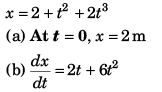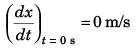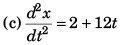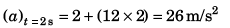Ques 22:  The velocity of a particle moving in a straight line is decreasing at the rate of 3 m/s per metre of displacement at an instant when the velocity is 10 m/s. Determine the acceleration of the particle at this instant.
Ans: - 30 ms-2
Sol: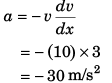Ques 23: The position o f a particle along a straight line is given by s = (t3 - 9t2 - 15t) m, here t is in seconds. Determine its maximum acceleration during the time interval 0 < / < 10s.
Ans:  42 ms-2
Sol: s = t3 - 9t2 - 15t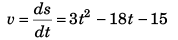ie,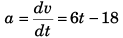Acceleration (a) in the interval 0 < t < 10 s will be maximum at t = 10s
a(at t = 10 s) = (6 x 10) - 18
= 42 m/s2

Ques 24: The acceleration of a particle is given by a (t) = (3.00 m/s2) - (2.00 m/s3)t.
(a) Find the initial speed v0 such that the particle will have the same x-coordinate at t = 5.00s as it had at t = 0.

(b) What will be the velocity at t = 5.00s?
Ans: (a) 0.833 ms-1
(b) - 9.17 ms-1
Sol: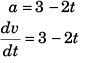or  ∫dv = ∫(3 - 2t) dt
or  v = 3t - t2 + c
or  v = 3t - t2 + v0 [as at t = 0, v = v0]
or   ∫ds = ∫ (3t - t2 + v0) dt
or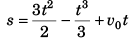(a) Displacement at = Displacement at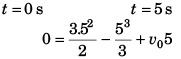⇒ v0 = 5/6 = 0.833 s
(b) v = 3 t - t2 + v0
Velocity at t (= 5.00 s)
= 3.5 - 52 + 0.833
= 15 - 25 + 833
= - 9.167 m/s

Ques 25: A particle moves along a horizontal path, such that its velocity is given by v = (3t2 - 6t) m/s, where t is the time in seconds. If it is initially located at the origin O, determine the distance travelled by the particle in time interval from t = 0 to t = 3.5s and the particle’s average velocity and average speed druing the same time interval.
Ans: 14.125 m, 1.75 ms-1, 4 .0 3 ms-1
Sol: v = 3t2 - 6t
∴ ∫ds = ∫(3t 2- 6t) dt
or  s = t3 - 3t2 + c
or   s = t3 - 3t2 = t2 (t - 3)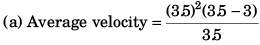= 3.5 x 0.5
= 1.75 m/s
(b) Distance covered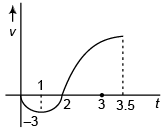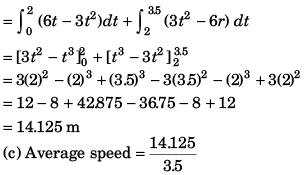= 4.036 m/s

Ques 26: A particle travels in a straight line, such that for a short time 2s < t < 6s, its motion is described by v = (4/a) m/s, where a is in m/s2. If v = 6 m/s when t = 2s, determine the particle’s acceleration when t = 3s.
Ans: 0.603 ms-2
Sol: v = 4/a
i.e.,     va = 4 …(i)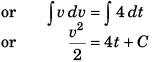Now, at t = 2 s, v = 6 m/s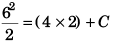Thus, C = 10.
i.e.,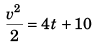…(ii)
∴   at     t = 3 s,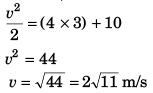Substituting above found value of v in Eq.
(i),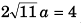i.e.,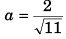= 0.603 m/s2

Ques 27: If the velocity v of a particle moving along a straight line decreases linearly with its displacement from 20 m/s to a value approaching zero at s = 30 m, determine the acceleration of the particle when s = 15 m.
Ans: (- 20/3) ms-2
Sol: According to question, the velocity of the particle varies as shown in figure.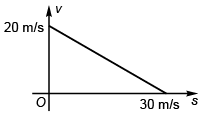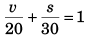or 3v + 2s = 60
or   3 v = 60 - 2s  …(i)
Differentiating above equation w.r.t. time t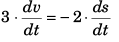or   3a = - 2v
or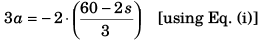or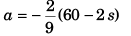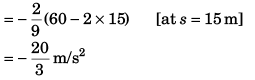The document Motion in One Dimension: JEE Main(Part- 2) - Physics, Solution by DC Pandey Notes | Study DC Pandey Solutions for JEE Physics - JEE is a part of the JEE Course DC Pandey Solutions for JEE Physics.
All you need of JEE at this link: JEEUse Code STAYHOME200 and get INR 200 additional OFF

## DC Pandey Solutions for JEE Physics

210 docs

Track your progress, build streaks, highlight & save important lessons and more!

,

,

,

,

,

,

,

,

,

,

,

,

,

,

,

,

,

,

,

,

,

,

,

,

;